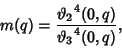## Nome

Given a Theta Function, the nome is defined as(1)

whereis the complete Elliptic Integral of the First Kind, andis the Parameter.(2)(3)

Solving the nome for the Parametergives(4)

whereis a Theta Function.

See also Amplitude, Characteristic (Elliptic Integral), Elliptic Integral, Modular Angle, Modulus (Elliptic Integral), Parameter

References

Abramowitz, M. and Stegun, C. A. (Eds.). Handbook of Mathematical Functions with Formulas, Graphs, and Mathematical Tables, 9th printing. New York: Dover, p. 591, 1972.